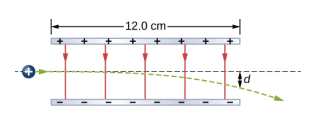# Electric Field Force Exerted Vertically

## Homework Statement

A proton enters the uniform electric field produced by the two charged plates shown below. The magnitude of the electric field is 4.0 × 105 N/C, and the speed of the proton when it enters is 1.5 × 107 m/s. What distance d has the proton been deflected downward when it leaves the plates?E = F/q
F = ma
PE = mgh
KE = 1/2mv^2

## The Attempt at a Solution

mgh = 1/2mv^2
gh = 1/2v^2
h = v^2 / 2g

Original height: h = (1.5*10^7)^2 / 2(9.8) = 1.15*10^13 m

E = F/q = ma/q
Eq / m = a
a = [(4*10^5) * (-1.6*10^-19)] / (1.673*10^-27)
a = -3.83 m/s^2

I'm not really sure where to go from here. Would I need time to get velocity from the acceleration I found? I'm just trying to find velocity for the proton with the force of the electric field acting on it and then using that in the height equation (which I'm not sure is the right one to use here) to find the difference between the original height where the proton goes straight forward (no electric field).

I also know the force of an electric field and can use its velocity to determine the time that the proton takes to travel through the 12 cm (or 12 * 10^-6 m) space but I have no idea how to compute the vertical distance it takes depending on the downward force exerted by the field.

#### Attachments

Last edited:

Doc Al
Mentor
I also know the force of an electric field and can use its velocity to determine the time that the proton takes to travel through the 12 cm (or 12 * 10^-6 m) space but I have no idea how to compute the vertical distance it takes depending on the downward force exerted by the field.
Use the given horizontal velocity to calculate the travel time through the field. Given the time and the acceleration that you calculated, you can apply basic kinematics to find the vertical distance.

Use the given horizontal velocity to calculate the travel time through the field. Given the time and the acceleration that you calculated, you can apply basic kinematics to find the vertical distance.

d = vt
t = d/v = (12*10^-2) / (1.5*10^7) = 8*10^-9s

Using kinematic y = yo + vo + 1/2at^2, I removed the yo and vo to get y = vo + 1/2at^2.
I first solved for the proton without the electric field
y = 1/2at^2
y = 1/2(9.8)(8*10^-9)^2 = 3.136*10^-16

Next, I solved for the proton with the field
y = 1/2at^2
y = 1/2(-3.83)(8*10^-9)^2 = -1.23*10^-16

So would the height difference be between 3.136*10^-16 and -1.23*10^-16? I'm not sure because these numbers look weird to me.

haruspex
Homework Helper
Gold Member
Using kinematic y = yo + vo + 1/2at^2
That is not dimensionally consistent. You cannot add a distance to a velocity. What have you left out?

•Civil_Disobedient
That is not dimensionally consistent. You cannot add a distance to a velocity. What have you left out?

That's just a basic kinematic equation, I may have incorrectly remembered it. I ended up using y=1/2at^2 to calculate height.

Doc Al
Mentor
E = F/q = ma/q
Eq / m = a
a = [(4*10^5) * (-1.6*10^-19)] / (1.673*10^-27)
a = -3.83 m/s^2
Right idea, but redo the arithmetic. (Watch those exponents.)

•Civil_Disobedient
Right idea, but redo the arithmetic. (Watch those exponents.)

Ah, I forgot to write in exponents for acceleration. a = 3.83*10^13. I also missed out how charge of a proton is positive, so acceleration would be positive.

So from there I recalculated y=1/2at^2
y = 1/2 * (3.83*10^13) * (8*10^-9) = 0.00123m

I'm a bit confused on finding the height difference, don't I also need to find height of the proton moving straight forward without the field? But when I do that, I still have 3.136*10^-16m.

Doc Al
Mentor
I'm a bit confused on finding the height difference, don't I also need to find height of the proton moving straight forward without the field?
The force that the field exerts on the proton is so much greater than its weight that you can safely ignore such a tiny correction due to gravity.

•Civil_Disobedient
The force that the field exerts on the proton is so much greater than its weight that you can safely ignore such a tiny correction due to gravity.

I see, that makes sense now. Thank you so much!

haruspex
•